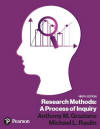﻿ The Results Section in APA Publication StyleGraziano & Raulin
Research Methods (9th edition)

## Results Section

The purpose of the results section is to tell the reader what was found in the study. A statistical description of the results is usually needed, as well as appropriate statistical tests.

Reporting statistical findings in a concise, yet understandable, way requires that the writer follow certain conventions. The usual convention is to present the descriptive statistics followed by the results of the inferential statistical tests. When reporting inferential statistics one should report what statistic was used, the number of degrees of freedom, the computed value of the statistic, and the probability of obtaining the computed value of the statistic by chance. The exact way of specifying various statistical results is shown in the section on statistics. With this format, readers can easily interpret the importance of certain results, even if they are not familiar with the statistical procedure used, because the probability value at the end of the report will mean the same thing. Anytime p is less than 0.05 (a traditional value of alpha), we would conclude the findings are unusual enough that it is unlikely they could have occurred as a result of chance.

Although it is important to express the statistical significance of comparisons made in the study, it is equally important to give the reader the information needed to interpret the results, such as the actual mean scores or frequencies.

Often the most effective way of presenting statistical information is to organize it in a table or figure. Tables and figures should be carefully labeled for the reader. In preparing a manuscript for publication, tables and figures are assembled and placed at the end of the manuscript. Each table or figure should be referred to in the manuscript.

There is no one correct way of presenting the results of a study. It is useful for the researcher to try to organize results in various way--testing both tables and figures to determine which method is most effective.